# L1_Functions_and_Relations.pptx

May. 20, 2023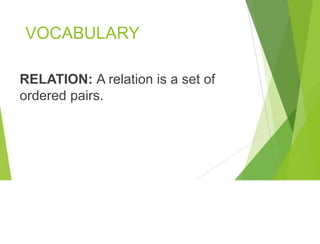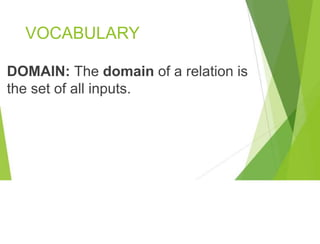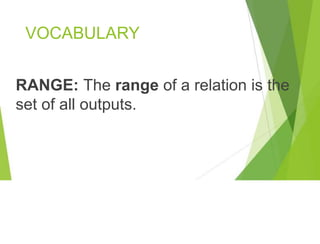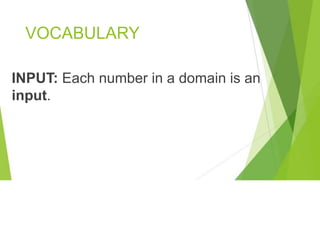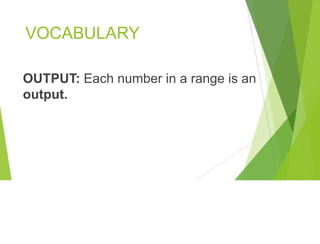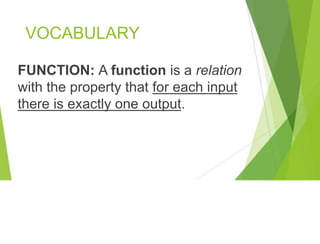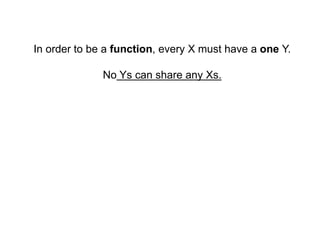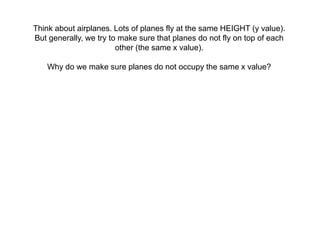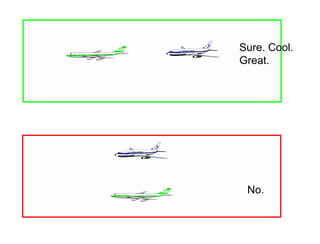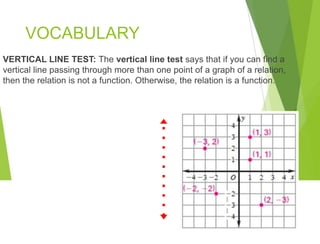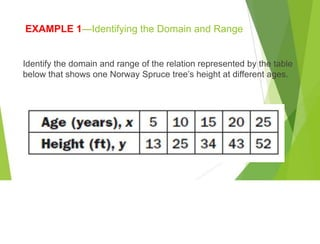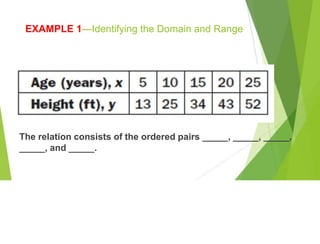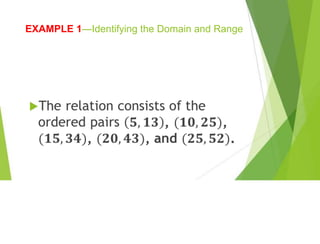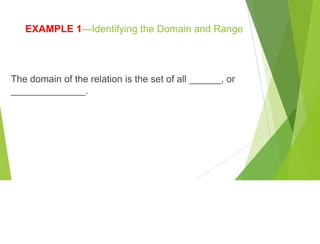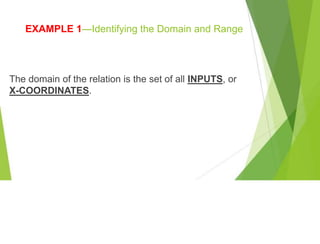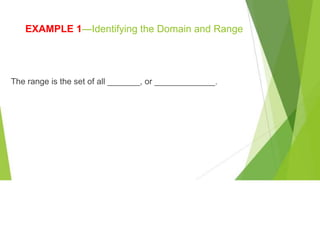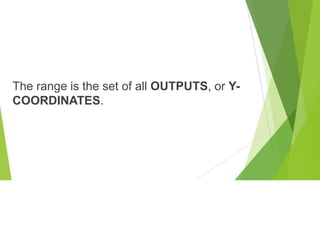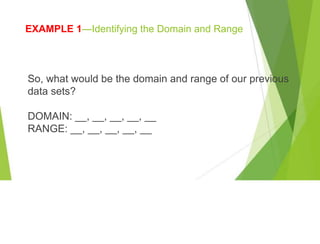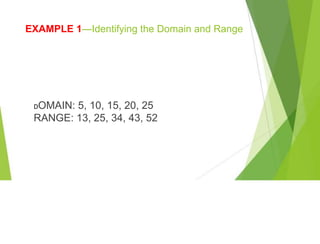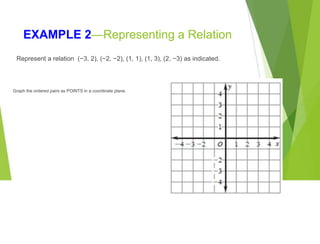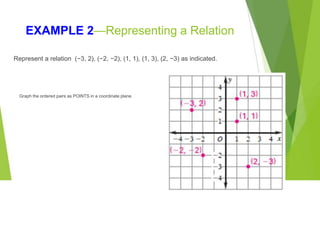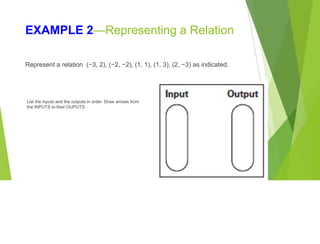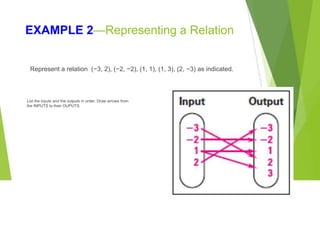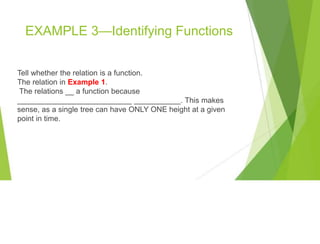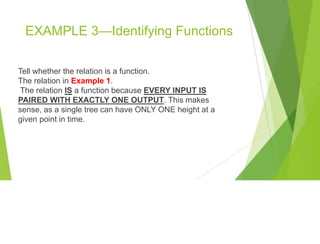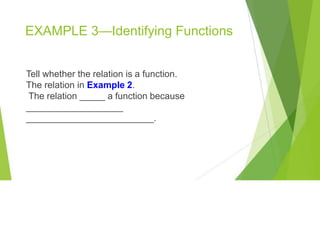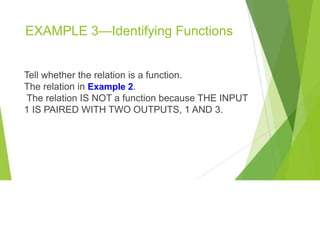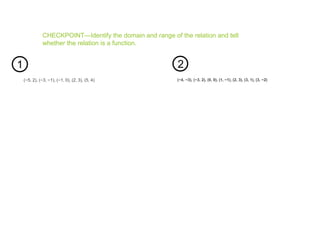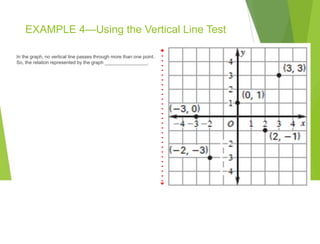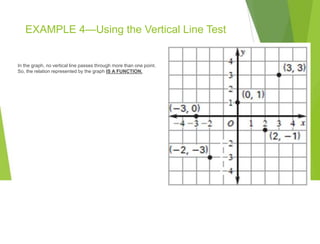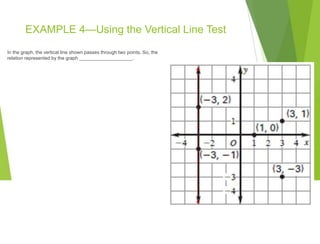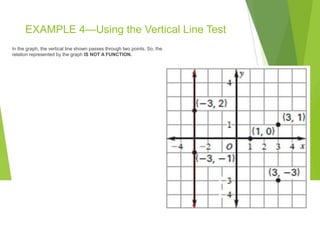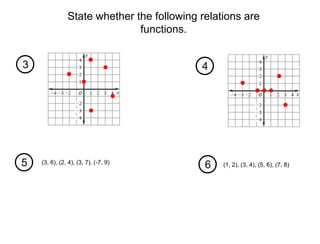1 of 35

### L1_Functions_and_Relations.pptx

• 2. VOCABULARY RELATION: A relation is a set of ordered pairs.
• 3. VOCABULARY DOMAIN: The domain of a relation is the set of all inputs.
• 4. VOCABULARY RANGE: The range of a relation is the set of all outputs.
• 5. VOCABULARY INPUT: Each number in a domain is an input.
• 6. VOCABULARY OUTPUT: Each number in a range is an output.
• 7. VOCABULARY FUNCTION: A function is a relation with the property that for each input there is exactly one output.
• 8. In order to be a function, every X must have a one Y. No Ys can share any Xs.
• 9. Think about airplanes. Lots of planes fly at the same HEIGHT (y value). But generally, we try to make sure that planes do not fly on top of each other (the same x value). Why do we make sure planes do not occupy the same x value?
• 11. VOCABULARY VERTICAL LINE TEST: The vertical line test says that if you can find a vertical line passing through more than one point of a graph of a relation, then the relation is not a function. Otherwise, the relation is a function.
• 12. EXAMPLE 1—Identifying the Domain and Range Identify the domain and range of the relation represented by the table below that shows one Norway Spruce tree’s height at different ages.
• 13. EXAMPLE 1—Identifying the Domain and Range The relation consists of the ordered pairs _____, _____, _____, _____, and _____.
• 14. EXAMPLE 1—Identifying the Domain and Range
• 15. EXAMPLE 1—Identifying the Domain and Range The domain of the relation is the set of all ______, or ______________.
• 16. EXAMPLE 1—Identifying the Domain and Range The domain of the relation is the set of all INPUTS, or X-COORDINATES.
• 17. EXAMPLE 1—Identifying the Domain and Range The range is the set of all _______, or _____________.
• 18. The range is the set of all OUTPUTS, or Y- COORDINATES.
• 19. EXAMPLE 1—Identifying the Domain and Range So, what would be the domain and range of our previous data sets? DOMAIN: __, __, __, __, __ RANGE: __, __, __, __, __
• 20. EXAMPLE 1—Identifying the Domain and Range DOMAIN: 5, 10, 15, 20, 25 RANGE: 13, 25, 34, 43, 52
• 21. EXAMPLE 2—Representing a Relation Represent a relation (−3, 2), (−2, −2), (1, 1), (1, 3), (2, −3) as indicated. Graph the ordered pairs as POINTS in a coordinate plane.
• 22. EXAMPLE 2—Representing a Relation Graph the ordered pairs as POINTS in a coordinate plane. Represent a relation (−3, 2), (−2, −2), (1, 1), (1, 3), (2, −3) as indicated.
• 23. EXAMPLE 2—Representing a Relation List the inputs and the outputs in order. Draw arrows from the INPUTS to their OUPUTS. Represent a relation (−3, 2), (−2, −2), (1, 1), (1, 3), (2, −3) as indicated.
• 24. EXAMPLE 2—Representing a Relation List the inputs and the outputs in order. Draw arrows from the INPUTS to their OUPUTS. Represent a relation (−3, 2), (−2, −2), (1, 1), (1, 3), (2, −3) as indicated.
• 25. EXAMPLE 3—Identifying Functions Tell whether the relation is a function. The relation in Example 1. The relations __ a function because ___________________________ ___________. This makes sense, as a single tree can have ONLY ONE height at a given point in time.
• 26. EXAMPLE 3—Identifying Functions Tell whether the relation is a function. The relation in Example 1. The relation IS a function because EVERY INPUT IS PAIRED WITH EXACTLY ONE OUTPUT. This makes sense, as a single tree can have ONLY ONE height at a given point in time.
• 27. EXAMPLE 3—Identifying Functions Tell whether the relation is a function. The relation in Example 2. The relation _____ a function because ___________________ _________________________.
• 28. EXAMPLE 3—Identifying Functions Tell whether the relation is a function. The relation in Example 2. The relation IS NOT a function because THE INPUT 1 IS PAIRED WITH TWO OUTPUTS, 1 AND 3.
• 29. CHECKPOINT—Identify the domain and range of the relation and tell whether the relation is a function. (−5, 2), (−3, −1), (−1, 0), (2, 3), (5, 4) (−4, −3), (−3, 2), (0, 0), (1, −1), (2, 3), (3, 1), (3, −2) 1 2
• 30. EXAMPLE 4—Using the Vertical Line Test In the graph, no vertical line passes through more than one point. So, the relation represented by the graph ________________.
• 31. EXAMPLE 4—Using the Vertical Line Test In the graph, no vertical line passes through more than one point. So, the relation represented by the graph IS A FUNCTION.
• 32. EXAMPLE 4—Using the Vertical Line Test In the graph, the vertical line shown passes through two points. So, the relation represented by the graph ____________________.
• 33. EXAMPLE 4—Using the Vertical Line Test In the graph, the vertical line shown passes through two points. So, the relation represented by the graph IS NOT A FUNCTION.
• 34. 3 State whether the following relations are functions. 4 5 6 (3, 6), (2, 4), (3, 7), (-7, 9) (1, 2), (3, 4), (5, 6), (7, 8)